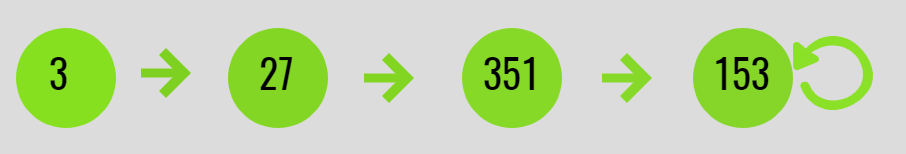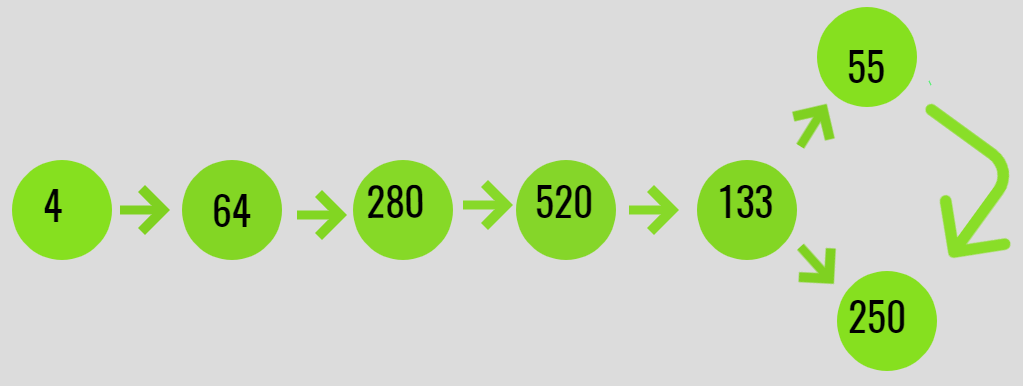GeeksforGeeks App
Open AppBrowser
Continue

# Check if digit cube limit of an integer arrives at fixed point or a limit cycle

Given an integer N, the task is to check if the Digit Cube limit of an integer arrives at a fixed point or in a limit cycle.

A Digit Cube Limit is a number which repeatedly arrives at a point if its value is computed as the sum of cubes of its digits, i.e. they have the following properties:

• Arrive at a fixed point if it is an Armstrong number.
• Arrive at a limit cycle if it is repeating in a cycle.

Examples:

Input: N = 3
Output: Reached to fixed point 153
Explanation:
F(3) = 3 * 3 * 3 = 27
F(27) = 2*2*2 + 7*7*7 = 351
F(351) = 3*3*3 + 5*5*5 + 1*1*1 = 153
F(153) = 1*1*1 + 5*5*5 + 3*3*3 = 153
Since a fixed point(= 153) was obtained, which is an Armstrong number of order 3. Below is the illustration:Input: N = 4
Output: Arrived at cycle
Explanation:
F(4) = 4 * 4 * 4 = 64
F(64) = 6 * 6 * 6 + 4 * 4 * 4 = 280
F(280) = 2 * 2 * 2 + 8 * 8 * 8 + 0 * 0 * 0 = 520
F(520) = 5 * 5 * 5 + 2 * 2 * 2 + 0*0*0 = 133
F(133) = 1*1*1 + 3*3*3 + 3*3*3 = 55
F(55) = 5*5*5 + 5*5*5 = 250
F(250) = 5*5*5 + 2*2*2 + 0*0*0 = 133
A cycle between
133 -> 55 -> 250 -> 133 is obtained.
Below is the illustration of the same:Approach: Follow the steps below to solve the problem:

• Create a hashMap to store the sum of the cube of digits of the number while iteration.
• Iterate for the next values for the sum of cube of digits of a number and Check if the next sum of the cube of digits is already present in the hashMap or not.
• If the number is already in the hash-map then, check if the number is an Armstrong number or not. If found to be true, then the number reaches a fixed point.
• Otherwise, If the number is not Armstrong number then continue.

Below is the implementation of the above approach:

## C++

 `// C++ program for the above approach` `#include ``#include ` `// Define the limit``#define limit 1000000000` `using` `namespace` `std;` `// Function to get the sum of cube``// of digits of a number``long` `long` `F(``long` `long` `N)``{``    ``// Convert to string to get sum``    ``// of the cubes of its digits``    ``string str = to_string(N);``    ``long` `long` `sum = 0;` `    ``for` `(``long` `long` `i = 0;``         ``i < str.size(); i++) {``        ``long` `long` `val``            ``= ``int``(str[i] - ``'0'``);``        ``sum += val * val * val;``    ``}` `    ``// return sum``    ``return` `sum;``}` `// Function to check if the number``// arrives at a fixed point or a cycle``long` `long` `findDestination(``long` `long` `N)``{``    ``// Stores the values obtained``    ``set<``long` `long``> s;` `    ``long` `long` `prev = N, next;` `    ``// Insert N to set s``    ``s.insert(N);` `    ``while` `(N <= limit) {` `        ``// Get the next number using F(N)``        ``next = F(N);` `        ``// Check if the next number is``        ``// repeated or not``        ``auto` `it = s.find(next);``        ``if` `(it != s.end()) {``            ``return` `next;``        ``}` `        ``prev = next;``        ``s.insert(prev);``        ``N = next;``    ``}``    ``return` `next;``}` `// Function to check if digit cube``// limit of an integer arrives at``// fixed point or in a limit cycle``void` `digitCubeLimit(``long` `long` `N)``{` `    ``// N is a non negative integer``    ``if` `(N < 0)``        ``cout << ``"N cannot be negative\n"``;` `    ``else` `{` `        ``// Function Call``        ``long` `long` `ans``            ``= findDestination(N);` `        ``// If the value received is``        ``// greater than limit``        ``if` `(ans > limit)``            ``cout << ``"Limit exceeded\n"``;` `        ``// If the value received is``        ``// an Armstrong number``        ``else` `if` `(ans == F(ans)) {``            ``cout << N;``            ``cout << ``" reaches to a"``                 ``<< ``" fixed point: "``;``            ``cout << ans;``        ``}` `        ``else` `{``            ``cout << N;``            ``cout << ``" reaches to a"``                 ``<< ``" limit cycle: "``;``            ``cout << ans;``        ``}``    ``}``}` `// Driver Code``int` `main()``{``    ``long` `long` `N = 3;` `    ``// Function Call``    ``digitCubeLimit(N);` `    ``return` `0;``}`

## Java

 `// Java program for the``// above approach``import` `java.util.*;``class` `GFG{``    ` `// Define the limit``static` `final` `int` `limit = ``1000000000``;``  ` `// Function to get the sum of cube``// of digits of a number``static` `int` `F(``int` `N)``{``  ``// Convert to String to get sum``  ``// of the cubes of its digits``  ``String str = String.valueOf(N);``  ``int` `sum = ``0``;` `  ``for` `(``int` `i = ``0``;``           ``i < str.length(); i++)``  ``{``    ``int` `val = (``int``)(str.charAt(i) - ``'0'``);``    ``sum += val * val * val;``  ``}` `  ``// return sum``  ``return` `sum;``}` `// Function to check if the number``// arrives at a fixed point or a cycle``static` `int` `findDestination(``int` `N)``{``  ``// Stores the values obtained``  ``HashSet s = ``new` `HashSet<>();` `  ``int` `prev = N, next =``0``;` `  ``// Insert N to set s``  ``s.add(N);` `  ``while` `(N <= limit)``  ``{``    ``// Get the next number``    ``// using F(N)``    ``next = F(N);` `    ``// Check if the next number is``    ``// repeated or not``    ``if` `(s.contains(next))``    ``{``      ``return` `next;``    ``}` `    ``prev = next;``    ``s.add(prev);``    ``N = next;``  ``}``  ``return` `next;``}` `// Function to check if digit cube``// limit of an integer arrives at``// fixed point or in a limit cycle``static` `void` `digitCubeLimit(``int` `N)``{``  ``// N is a non negative integer``  ``if` `(N < ``0``)``    ``System.out.print(``"N cannot be negative\n"``);``  ``else``  ``{``    ``// Function Call``    ``int` `ans = findDestination(N);` `    ``// If the value received is``    ``// greater than limit``    ``if` `(ans > limit)``      ``System.out.print(``"Limit exceeded\n"``);` `    ``// If the value received is``    ``// an Armstrong number``    ``else` `if` `(ans == F(ans))``    ``{``      ``System.out.print(N);``      ``System.out.print(``" reaches to a"` `+``                       ``" fixed point: "``);``      ``System.out.print(ans);``    ``}` `    ``else``    ``{``      ``System.out.print(N);``      ``System.out.print(``" reaches to a"` `+``                       ``" limit cycle: "``);``      ``System.out.print(ans);``    ``}``  ``}``}` `// Driver Code``public` `static` `void` `main(String[] args)``{``  ``int` `N = ``3``;` `  ``// Function Call``  ``digitCubeLimit(N);``}``}` `// This code is contributed by Rajput-Ji`

## Python3

 `# Python3 program for the above approach` `# Define the limit``LIMIT ``=` `1000000000` `# Function to get the sum of cube``# of digits of a number``def` `F(N: ``int``) ``-``> ``int``:``    ` `    ``# Convert to string to get sum``    ``# of the cubes of its digits``    ``string ``=` `str``(N)``    ``sum` `=` `0``    ` `    ``for` `i ``in` `range``(``len``(string)):``        ``val ``=` `int``(``ord``(string[i]) ``-` `ord``(``'0'``))``        ``sum` `+``=` `val ``*` `val ``*` `val` `    ``# Return sum``    ``return` `sum` `# Function to check if the number``# arrives at a fixed point or a cycle``def` `findDestination(N: ``int``) ``-``> ``int``:``    ` `    ``# Stores the values obtained``    ``s ``=` `set``()` `    ``prev ``=` `N``    ``next` `=` `0` `    ``# Insert N to set s``    ``s.add(N)` `    ``while` `(N <``=` `LIMIT):` `        ``# Get the next number using F(N)``        ``next` `=` `F(N)` `        ``# Check if the next number is``        ``# repeated or not``        ``if` `next` `in` `s:``            ``return` `next` `        ``prev ``=` `next``        ``s.add(prev)``        ``N ``=` `next` `    ``return` `next` `# Function to check if digit cube``# limit of an integer arrives at``# fixed point or in a limit cycle``def` `digitCubeLimit(N: ``int``) ``-``> ``int``:``    ` `    ``# N is a non negative integer``    ``if` `(N < ``0``):``        ``print``(``"N cannot be negative"``)` `    ``else``:` `        ``# Function Call``        ``ans ``=` `findDestination(N)` `        ``# If the value received is``        ``# greater than limit``        ``if` `(ans > LIMIT):``            ``print``(``"Limit exceeded"``)` `        ``# If the value received is``        ``# an Armstrong number``        ``elif` `(ans ``=``=` `F(ans)):``            ``print``(``"{} reaches to a fixed point: {}"``.``format``(``                ``N, ans))``        ``else``:``            ``print``(``"{} reaches to a limit cycle: {}"``.``format``(``                ``N, ans))` `# Driver Code``if` `__name__ ``=``=` `"__main__"``:``    ` `    ``N ``=` `3` `    ``# Function Call``    ``digitCubeLimit(N)` `# This code is contributed by sanjeev2552`

## C#

 `// C# program for the``// above approach``using` `System;``using` `System.Collections.Generic;``class` `GFG{``    ` `// Define the limit``static` `readonly` `int` `limit =``       ``1000000000;``  ` `// Function to get the sum``// of cube of digits of a``// number``static` `int` `F(``int` `N)``{``  ``// Convert to String to get sum``  ``// of the cubes of its digits``  ``String str = String.Join(``""``, N);``  ``int` `sum = 0;` `  ``for` `(``int` `i = 0;``           ``i < str.Length; i++)``  ``{``    ``int` `val = (``int``)(str[i] - ``'0'``);``    ``sum += val * val * val;``  ``}` `  ``// return sum``  ``return` `sum;``}` `// Function to check if the``// number arrives at a fixed``// point or a cycle``static` `int` `findDestination(``int` `N)``{``  ``// Stores the values``  ``// obtained``  ``HashSet<``int``> s =``          ``new` `HashSet<``int``>();` `  ``int` `prev = N, next = 0;` `  ``// Insert N to set s``  ``s.Add(N);` `  ``while` `(N <= limit)``  ``{``    ``// Get the next number``    ``// using F(N)``    ``next = F(N);` `    ``// Check if the next``    ``// number is repeated``    ``// or not``    ``if` `(s.Contains(next))``    ``{``      ``return` `next;``    ``}` `    ``prev = next;``    ``s.Add(prev);``    ``N = next;``  ``}``  ``return` `next;``}` `// Function to check if digit cube``// limit of an integer arrives at``// fixed point or in a limit cycle``static` `void` `digitCubeLimit(``int` `N)``{``  ``// N is a non negative integer``  ``if` `(N < 0)``    ``Console.Write(``"N cannot be negative\n"``);``  ``else``  ``{``    ``// Function Call``    ``int` `ans = findDestination(N);` `    ``// If the value received is``    ``// greater than limit``    ``if` `(ans > limit)``      ``Console.Write(``"Limit exceeded\n"``);` `    ``// If the value received is``    ``// an Armstrong number``    ``else` `if` `(ans == F(ans))``    ``{``      ``Console.Write(N);``      ``Console.Write(``" reaches to a"` `+``                    ``" fixed point: "``);``      ``Console.Write(ans);``    ``}` `    ``else``    ``{``      ``Console.Write(N);``      ``Console.Write(``" reaches to a"` `+``                    ``" limit cycle: "``);``      ``Console.Write(ans);``    ``}``  ``}``}` `// Driver Code``public` `static` `void` `Main(String[] args)``{``  ``int` `N = 3;` `  ``// Function Call``  ``digitCubeLimit(N);``}``}` `// This code is contributed by gauravrajput1`

## Javascript

 ``

Output:

`3 reaches to a fixed point: 153`

Time Complexity: O(N)
Auxiliary Space: O(N)

My Personal Notes arrow_drop_up